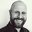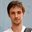Question-and-Answer Resource for the Building Energy Modeling Community
Get started with the Help page

# How is Site Standard Air Density calculated in EnergyPlus?In EnergyPlus documentation, I did not find any description about how the site elevation corrected standard air density is calculated. I assume it follows the method described in the ASHRAE HOF, just want to confirm.

edit retag close merge delete

Sort by » oldest newest most votedIt would appear that you're correct. According to the DesignBuilder help, it follows this approach:

Air is assumed to be dry and at 20°C. The site elevation is used to calculate standard atmospheric pressure using the equation for 'standard atmospheric' pressure on p 6.1 of the ASHRAE 1997 HOF.

Density = P / (R * T)

Where:

R is the Gas constant = 287.05 J/kg-K

T is the temperature in K. Air is assumed to be at 20°C so, T = (20 + 273.15)

P is standard pressure:

P = 101325 x (1.0 − Z x 0.0000225577)^5.2559

where:

Z = Elevation above sea level (m)

This is referred to in the Engineering Reference section on demand controlled ventilation.

RhoStd = standard air density (1.204 kg/m${^3}$}) adjusted for the local barometric pressure (standard barometric pressure corrected for altitude, ASHRAE 1997 HOF pg. 6.1).

more